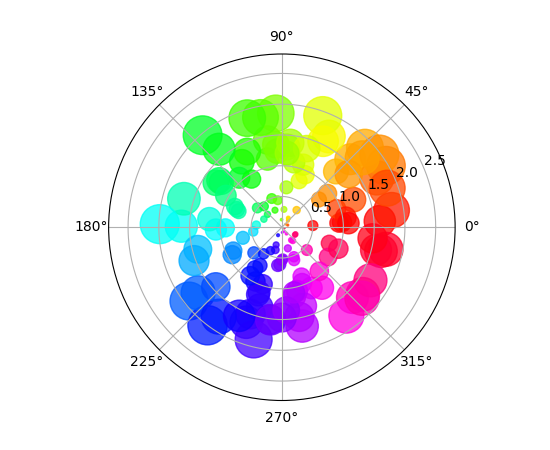Travis-CI:# pie_and_polar_charts example code: polar_scatter_demo.py¶```"""
==========================
Scatter plot on polar axis
==========================

Demo of scatter plot on a polar axis.

Size increases radially in this example and color increases with angle
(just to verify the symbols are being scattered correctly).
"""
import numpy as np
import matplotlib.pyplot as plt

# Compute areas and colors
N = 150
r = 2 * np.random.rand(N)
theta = 2 * np.pi * np.random.rand(N)
area = 200 * r**2
colors = theta

ax = plt.subplot(111, projection='polar')
c = ax.scatter(theta, r, c=colors, s=area, cmap='hsv', alpha=0.75)

plt.show()
```

Keywords: python, matplotlib, pylab, example, codex (see Search examples)